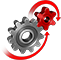# WolframSystemModeler

## Tennis Racket Theorem (Dzhanibekov Effect)

The tennis racket theorem or the Dzhanibekov effect states that for a rigid body with three unique moments of inertia, rotation about the intermediate axis is unstable, while rotation about the other two axes is stable. You can see this theorem in action in this wing nut example.

To run this example, you'll need

### The Model

Import or create a CAD model of the wing nut and create a SystemModel with the CAD data (including the physical properties).Use CreateSystemModel functionality to create SystemModels.

### Visualization

The wing nut is initialized with a linear velocity and an angular velocity in a zero gravity environment. You can see in the following video that the wing nut flips during its motion.

Instant animation

Automatically create 3D animations for models.

Demonstration of tennis racket theorem using a wing nut.

Programmatically create models